FAIL (the browser should render some flash content, not this).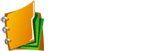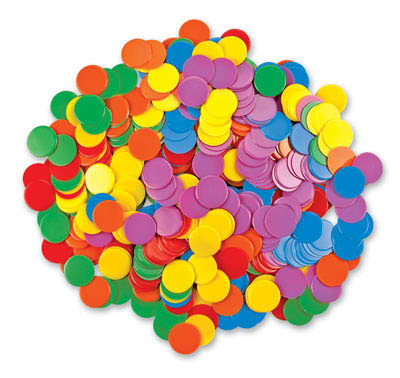Math Challengers Ch 1 LESSONS

HW

SOL

PDF
PDF

PDF
PDF
PDF

How to Count without Counting

In this chapter, students will learn:

• different strategies of counting
• combinations and permutations
Quiz #1 Counting Principles
Quiz #2 Permutations and Counting
• Quiz on Permutations
• Difficult Counting Quiz Math ChallengersMath Challengers Ch 2
HW

PDF
PDF
PDF
PDF
PDF
PDF

SOL

PDF
PDF
PDF
PDF
PDF
PDF

Probability and Chances

In this chapter, students will learn:

• the basics to understanding probability
• how to create a sample space
• recognizing different cases in probability problems
• Proability Quiz #1
• Probability Quiz Difficult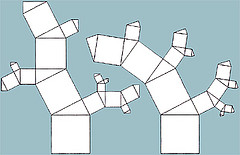Math Challengers Ch 3

•  Areas of Triangles
•  Pythagorean Theorem and Triples
•  Special Triangles
•  Similiar Triangles
•  Median, Altitude, and Properties of Triangles

PDF
PDF
PDF
PDF
PDF
Properties of Triangles

In this chapter, students will learn:

• to develop and apply the Pythagorean Theorem
• all the properties of triangles
• how to find areas using similiar triangles
• Heron's formula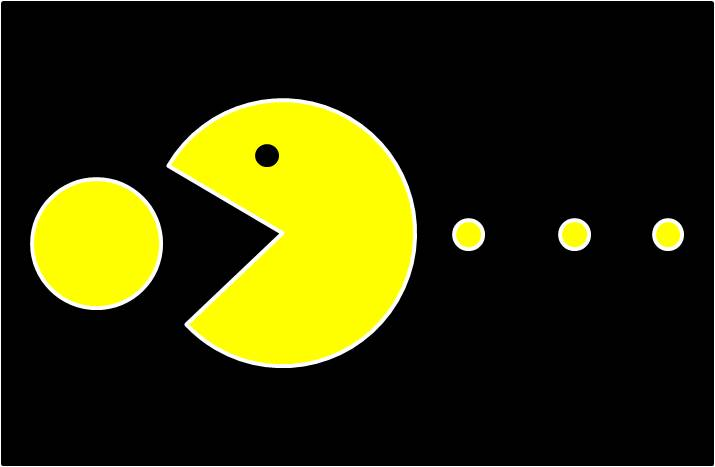Math Challengers Ch 4

•  Problems Involving Circles and Semi Circles
•  Problems Involving Regular Solids

PDF

PDF

Areas of 2D Figures

In this section, students will learn:

• solve problems involving circles and other 2D shapes
• recognize patterns of regular solidsMath Challengers Ch 5

•  Challenge Problems on Circle Geometry

PDF
PDF
PDF
PDF
PDF
Angles and Geometry

In this section, students will learn:
• to solve problems involving angles and circles
• find angles involving parallel lines, tangents, and secants
• properties of circle geometry
• Quiz 5.1: Angles with Parallel Lines and Triangles
• Quiz 5.2 Chord Properties in Circles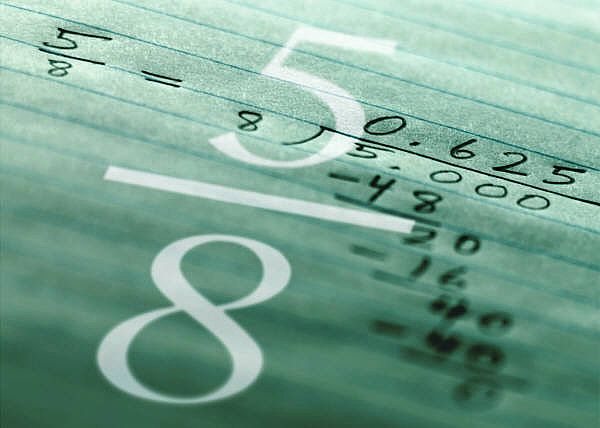Math Challengers Ch 6

•  Function Notations
•  Composite Functions
•  Sequences and Series
•  Recursive Functions

PDF
PDF
PDF
PDF
PDF

Functions and Formulas

In this section, students will learn:

• the operations of various functions
• how to calculate the output of a function
• functions of recursive sequencesMath Challengers Ch 7

•  Problems Involving Rates

•  Problems Involving Ratios

PDF

PDF

Rates and Ratios

In this section, students will learn:

• to solve challenging problems involving rates and ratiosMath Challengers Ch 8

•  Volumes of Basic Solids
•  Areas of Basic Solids
•  Volumes of Composite Solids
•  Challenge Problems with Solids

PDF
PDF
PDF
PDF
Areas and Volumes of 3D Solids

In this section, students will learn:

• to find the volume and surface areas of composite solids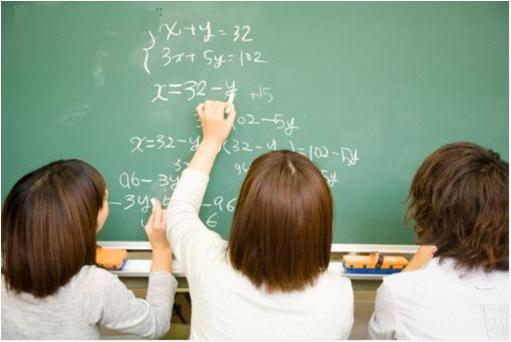Math Count Countdown Rounds

PDF
PDF
PDF
PDF
Practice Materials

•  2008 Intramurals Target
•  2008 Intramurals Team
•  Challenge Problems with SolidsMath Challengers Resources

PDF
PDF
PDF
PDF
Practice Materials

•  Math Count Past Contests
•  Math Counts Workbooks
•  95 Problems of the Day
•  Math Count Test Taking Page
•  Math Count Past Contests
•  Math Count Past Contests